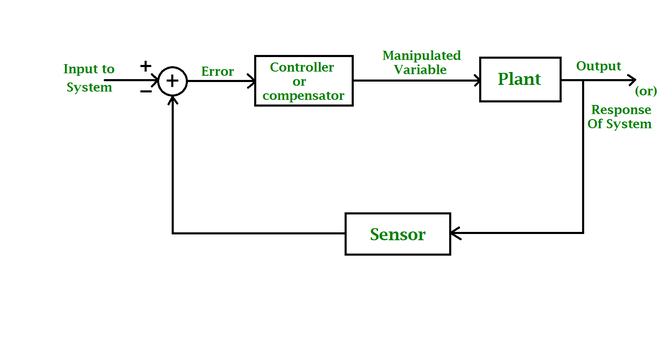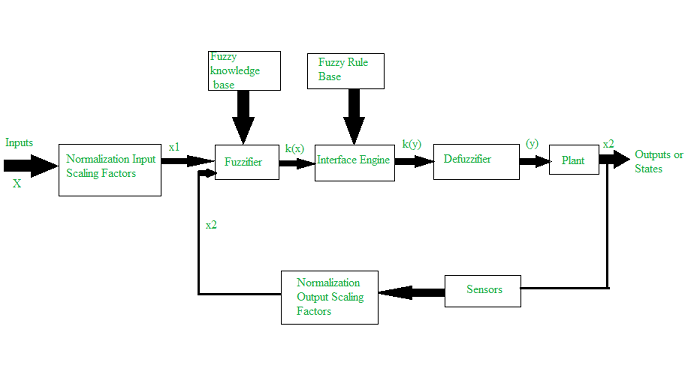Skip to content
Related Articles
Fuzzy Logic Control System
• Last Updated : 26 Nov, 2020

Introduction:

Fuzzy logic control (FLC) is the most active research area in the application of fuzzy set theory, fuzzy reasoning, and fuzzy logic. The application of FLC extends from industrial process control to biomedical instrumentation and securities. Compared to conventional control techniques, FLC has been best utilized in complex ill-defined problems, which can be controlled by an efficient human operator without knowledge of their underlying dynamics.

A control system is an arrangement of physical components designed to alter another physical system so that this system exhibits certain desired characteristics. There exist two types of control systems: open-loop and closed-loop control systems. In open-loop control systems, the input control action is independent of the physical system output. On the other hand, in a closed-loop control system, the input control action depends on the physical system output. Closed-Hoop control systems are also known as feedback control systems. The first step toward controlling any physical variable is to measure it. A sensor measures the controlled signal, A plant is a physical system under control. In a closed-loop control system, forcing signals of the system inputs are determined by the output responses of the system. The basic control problem is given as follows:

The output of the physical system under control is adjusted by the help of an error signal. The difference between the actual response (calculated) of the płant and the desired response gives the error signal. For obtaining satisfactory responses and characteristics for the closed-loop control system, an additional system, called as compensator or controller, can be added to the loop. The basic block diagram of the closed-loop control system is shown in Figure 1. The fuzzy control rules are basically IE-THEN rules.Fig 1: Block Diagram of closed-loop Control System

Control System Design:

Designing a controller for a complex physical system involves the following steps:

1. Decomposing the large-scale system into a collection of various subsystems.
2. Varying the plant dynamics slowly and linearizing the nonlinear plane dynamics about a set of operating points.
3. Organizing a set of state variables, control variables, or output features for the system under consideration.
4. 4. Designing simple P, PD, PID controllers for the subsystems. Optimal controllers can also be designed.

Apart from the first four steps, there may be uncertainties occurring due to external environmental conditions. The design of the controller should be made as dose as possible to the optimal controller design based on the expert knowledge of the control engineer. This may be done by various numerical observations of the input-output relationship in the form of linguistic, intuitive, and other kinds of related information related to the dynamics of the plant and the external environment. Finally, a supervisory control system, either manual operator or automatic, forms an extra feedback control loop to tune and adjust the parameters of the controller, for compensating the variational effects caused by nonlinear and remodelled dynamics. In comparison with a conventional control system design, an FLC system design should have the following assumptions made, in case it is selected. The plant under consideration should be observable and controllable. A wide range of knowledge comprising a set of expert linguistic rules, basic engineering common sense, a set of data for input/output, or a controller analytic model, which can be fuzzified and from which the fuzzy rule the base can be formed, should exist. Also, for the problem under consideration, a solution should exist and it should be such that the control the engineer is working for a “good” solution and not especially looking for an optimum solution. The controller, in this case, should be designed to the best of our ability and within an acceptable range of precision. It should be noted that the problems of stability and optimality are ongoing problems in the fuzzy controller design.

In designing a fuzzy logic controller, the process of forming fuzzy rules plays a vital role. There are four structures of the fuzzy production rule system (Weiss and Donnel, 1979) which are as follows:

1. A set of rules that represents the policies and heuristic strategies of the expert decision-maker.
2. A set of input data that are assessed immediately prior to the actual decision.
3. A method for evaluating any proposed action in terms of its conformity to the expressed rules when there is available data.
4. A method for generating promising actions and determining when to stop searching for better ones.

All the necessary parameters used in the fuzzy logic controller are defined by membership functions. The rules are evaluated using techniques such as approximate reasoning or interpolative reasoning. These four structures of fuzzy rules help in obtaining the control surface that relates the control action to the measured state or output variable. The control surface can then be sampled down to a finite number of points and based on this information, a look~up table may be Constructed. The look~up table comprises the information about the control surface which can be downloaded into a read·only memory chip. This chip would constitute a fixed controller for the plant.

Architecture and Operations of FLC System:

The basic architecture of a fuzzy logic controller is shown in Figure 2. The principal components of an FLC system is a fuzzifier, a fuzzy rule base, a fuzzy knowledge base, an inference engine, and a defuzz.ifier. It also includes parameters for normalization. When the output from the defuzzifier is not a control action for a plant, then the system is a fuzzy logic decision system. The fuzzifier present converts crisp quantities into fuzzy quantities. The fuzzy rule base stores knowledge about the operation of the process of domain expertise. The fuzzy knowledge base stores the knowledge about all the input-output fuzzy relationships. It includes the membership functions defining the input variables to the fuzzy rule base and the out variables to the plant under control. The inference engine is the kernel of an FLC system, and it possesses the capability to simulate human decisions by performing approximate reasoning to achieve the desired control strategy. The defuzzifier converts the fuzzy quantities into crisp quantities from an inferred fuzzy control action by the inference engine.Fig 2: Basic architecture of a FLC System

The various steps involved in designing a fuzzy logic controller are as follows:

• Step 1: Locate the input, output, and state variables of the plane under consideration. I
• Step 2: Split the complete universe of discourse spanned by each variable into a number of fuzzy subsets, assigning each with a linguistic label. The subsets include all the elements in the universe.
• Step 3: Obtain the membership function for each fuzzy subset.
• Step 4: Assign the fuzzy relationships between the inputs or states of fuzzy subsets on one side and the output of fuzzy subsets on the other side, thereby forming the rule base.
• Step 5: Choose appropriate scaling factors for the input and output variables for normalizing the variables between [0, 1] and [-1, I] interval.
• Step 6: Carry out the fuzzification process.
• Step 7: Identify the output contributed from each rule using fuzzy approximate reasoning.
• Step 8: Combine the fuzzy outputs obtained from each rule.
• Step 9: Finally, apply defuzzification to form a crisp output.

The above steps are performed and executed for a simple FLC system. The following design elements are adopted for designing a general FLC system:

1. Fuzzification strategies and the interpretation of a fuzzifier.
2. Fuzzy knowledge base: Normalization of the parameters involved; partitioning of input and output spaces; selection of membership functions of a primary fuzzy set.
3. Fuzzy rule base: Selection of input and output variables; the source from which fuzzy control rules are to be derived; types of fuzzy control rules; completeness of fuzzy control rules.
4. Decision· making logic: The proper definition of fuzzy implication; interpretation of connective “and”; interpretation of connective “or”; inference engine.
5. Defuzzification materials and the interpretation of a defuzzifier.

Applications:

FLC systems find a wide range of applications in various industrial and commercial products and systems. In several applications- related to nonlinear, time-varying, ill-defined systems and also complex systems – FLC systems have proved to be very efficient in comparison with other conventional control systems. The applications of FLC systems include:

1. Traffic Control
2. Steam Engine
3. Aircraft Flight Control
4. Missile Control
5. Adaptive Control
6. Liquid-Level Control
7. Helicopter Model
8. Automobile Speed Controller
9. Braking System Controller
10. Process Control (includes cement kiln control)
11. Robotic Control
12. Elevator (Automatic Lift) control;
13. Automatic Running Control
14. Cooling Plant Control
15. Water Treatment
16. Boiler Control;
17. Nuclear Reactor Control;
18. Power Systems Control;
19. Air Conditioner Control (Temperature Controller)
20. Biological Processes
21. Knowledge-Based System
22. Fault Detection Control Unit
23. Fuzzy Hardware implementation and Fuzzy ComputersMy Personal Notes arrow_drop_up